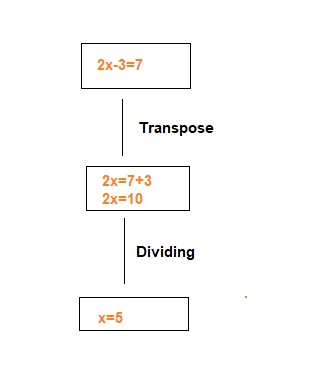< NCERT Solutions for Class 8 Maths Chapter 2 Linear equations in One Variable Exercise 2.1# NCERT Solutions for Class 8 Maths Chapter 2 Linear equations in One Variable Exercise 2.1

In this page we have NCERT Solutions for Class 8 Maths Chapter 2 Linear equations in One Variable for Exercise 2.1.This exercise has questions about simple linear equation where we have linear expression on one side and Numbers on Other side .the Hope you like them and do not forget to like , social share and comment at the end of the page.
Solving Equations which have Linear Expressions on one Side and Numbers on the other Side## NCERT Solutions for Class 8 Maths Chapter 2 Exercise 2.1

Question 1:
Solve: x-2=7
x - 2 = 7
Transposing 2 to R.H.S, we obtain
x = 7 + 2 = 9

Question 2:
Solve: y+3=10
y + 3 = 10
Transposing 3 to R.H.S, we obtain
y = 10 - 3 = 7

Question 3:
Solve: 6=z+2
6=z+2
Or
z+2=6
Transposing 2 to R.H.S, we obtain
z=6 -2=4

Question 4
Solve:
$\frac {3}{7} + x =\frac {17}{7}$

$\frac {3}{7} + x =\frac {17}{7}$
Multiplying 7 on both sides
3+7x=17
Transposing 3 to R.H.S, we obtain
7x=17-3=14
7x=14
Dividing both the sides by 7
x=2

Question 5:
Solve:
6x=12

6x=12
Dividing both the sides by 6
x=2

Question 6:
Solve:
$\frac {t}{5}=10$
$\frac {t}{5}=10$
Multiplying 5 on both sides
t=50

Question 7:
Solve
$\frac {2x}{3}=18$
$\frac {2x}{3}=18$
Multiplying both the sides by 3
2x=54
Dividing both the sides by 2
x=27

Question 8:
Solve:
$1.6 = \frac {y}{1.5}$

$1.6 = \frac {y}{1.5}$
Multiplying both the sides by 1.5
1.6 × 1.5=y
y=2.4

Question 9:
Solve:
7x-9=16
7x-9=16
Transposing 9 to R.H.S, we obtain
7x=16+9
7x=25
Dividing by 7 on both the sides
x=25/7

Question 10:
Solve:
14y-8=13

14y-8=13
Transposing 8 to R.H.S, we obtain
14y=13+8
14y=21
Dividing 14 on both the sides
y=21/14=3/2

Question 11:
Solve:
17+6p=9
17+6p=9
Transposing 17 to R.H.S, we obtain
6p=9-17
6p=-8
Dividing 6 on both the sides
p=-8/6=-4/3

Question 12:
Solve:
$\frac {x}{3}+1=\frac {7}{15}$

$\frac {x}{3}+1=\frac {7}{15}$
The LCM of denominator is 15, So multiplying both sides by 15
5x +15=7
Transposing 15 to R.H.S, we obtain
5x=-8
Dividing 5 on both the sides
x=-8/5

## Summary

1. NCERT Solutions for Class 8 Maths Chapter 2 Linear equations in One Variable Exercise 2.1 has been prepared by Expert with utmost care. If you find any mistake.Please do provide feedback on mail. You can download the solutions as PDF in the below Link also
2. This chapter 2 has total 6 Exercise 2.1,2.2,2.3,2.4,2.5.2.6. This is the First exercise in the chapter.You can explore previous exercise of this chapter by clicking the link below